﻿ 导管桨内流场及涡特性DES模拟
«上一篇文章快速检索 高级检索

 哈尔滨工程大学学报2019, Vol. 40Issue (8): 1381-1386  DOI: 10.11990/jheu.2018071190

引用本文GONG Jie, GUO Chunyu, ZHAO Dagang, et al. Detached eddy simulations of internal flow fields and vortex characteristics of ducted propellers[J]. Journal of Harbin Engineering University, 2019, 40(8), 1381-1386. DOI: 10.11990/jheu.201807119.文章历史

1. 哈尔滨工程大学 船舶工程学院, 黑龙江 哈尔滨 150001;
2. 海洋石油工程股份有限公司, 天津 300384

Detached eddy simulations of internal flow fields and vortex characteristics of ducted propellers
GONG Jie 1, GUO Chunyu 1, ZHAO Dagang 1, SONG Kewei 1, ZHONG Wenjun 2
1. College of Shipbuilding Engineering, Harbin Engineering University, Harbin 150001, China;
2. Offshore Oil Engineering Co. Ltd., Tianjin 300384, China
Abstract: The internal flow fields and vortex characteristics of a ducted propeller are simulated by using the detached eddy simulation (DES) method to analyze the internal flow of ducted propellers and the components and distribution of vortex structures. Numerical simulations are performed as unsteady, three-dimensional, viscous, and incompressible calculations. Sliding mesh technology is employed in the open-water test simulation, and the volume-rendering method is used to present the spatial structures of internal vortices. The numerical results for hydrodynamic performance are in good agreement with the model test results. Results show that the flow near the inner surface of the duct is complex, and the peak values of surface pressure fluctuation occur continuously in the blade-passing frequency and its multiples in the frequency domain. The DES model can effectively simulate different ducted propeller vortex structures, such as duct shear-layer, blade-tip, blade-shedding, and blade-root vortices. Changes in tip vortex morphology and vorticity distribution cover a large area of the inner surface of the duct. All of the above factors contribute to the recovery of wake energy and improve propulsion efficiency.
Keywords: ducted propeller    internal flow field    detached eddy simulation    pressure fluctuation    vortex structure

1 几何模型与计算区域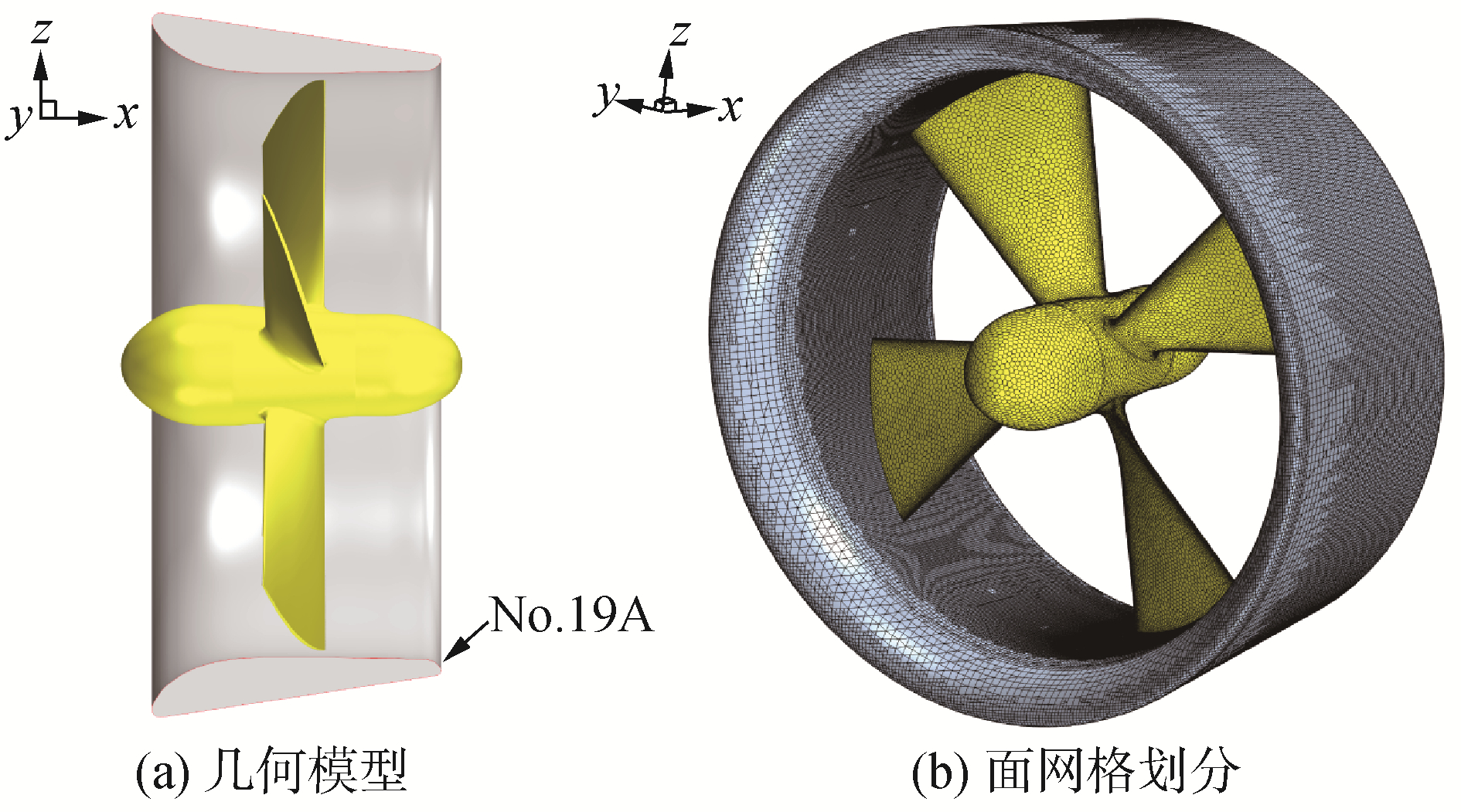Download: 图 1 导管桨几何模型与网格划分 Fig. 1 Geometry model and mesh generation of ducted propeller

2 数值模型建立 2.1 湍流模型与离散方法

 $\nu_{t}=\tilde{\nu} f_{\nu 1}$ (1)
 $f_{\nu 1}=\chi^{3} /\left(\chi^{3}+C_{\nu 1}^{3}\right)$ (2)
 $\chi=\tilde{\nu} / \nu$ (3)

 $\begin{array}{c}{\frac{\partial \tilde{\nu}}{\partial t}+u_{j} \frac{\partial \tilde{\nu}}{\partial x_{j}}=\frac{1}{\sigma}\{\nabla \cdot[(\nu+\tilde{\nu}) \nabla \tilde{\nu}]+} \\ {C_{b 2}\left|\nabla_{\nu}\right|^{2} \}+C_{b 1}\left[1-f_{t 2}\right] \tilde{S} \tilde{\nu}-} \\ {\left[C_{w 1} f_{w}-\frac{C_{b 1}}{\kappa^{2}} f_{l 2}\right]\left(\frac{\tilde{\nu}}{d}\right)^{2}+f_{t 1} \Delta U^{2}}\end{array}$ (4)

 $\tilde{d}=\min \left(d, C_{\mathrm{DES}} \mathit{\Delta}\right)$ (5)

DES模拟采用SIMPLE算法完成压力-速度耦合方程的求解。其中，对流项通过二阶迎风格式进行离散，扩散项通过中心差分格式进行离散，时间项二阶隐式格式离散，均采用Spalart-Allmaras湍流模型封闭N-S方程组。

2.2 网格划分

3 计算结果与讨论 3.1 敞水性能计算结果验证

 $\left\{\begin{array}{l}{J=V_{x} / n D} \\ {K_{t p}=T_{p} / \rho n^{2} D^{4}} \\ {K_{t d}=T_{d} / \rho n^{2} D^{4}} \\ {K_{q}=Q_{0} / \rho n^{2} D^{5}} \\ {\eta_{0}=\frac{J}{2 \pi} \frac{K_{t p}+K_{t d}}{K_{q}}}\end{array}\right.$ (6)表 1 导管桨数值计算与模型试验结果对比 Table 1 Comparison of numerical calculation and model test results of ducted propeller
3.2 内流场特性分析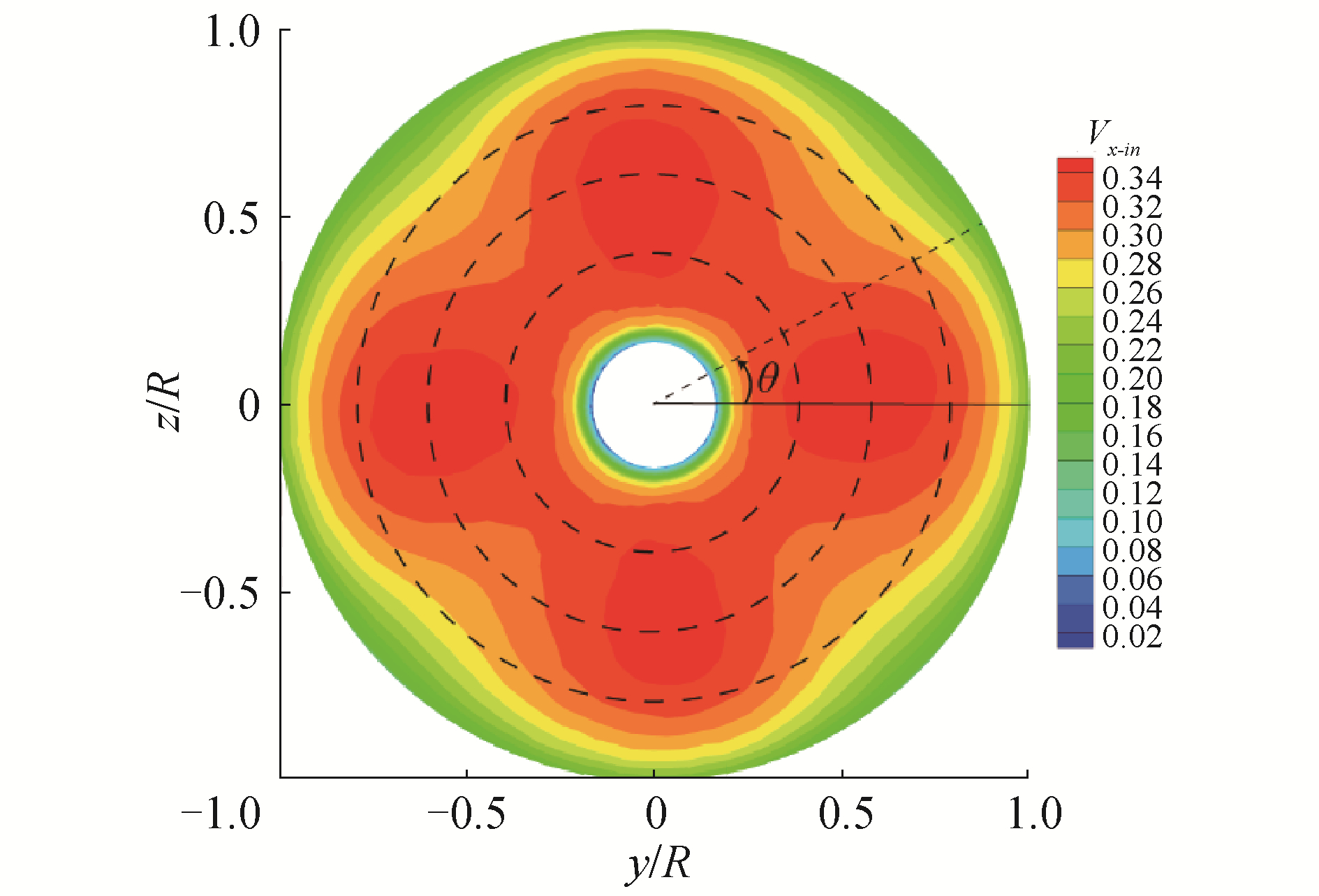Download: 图 2 桨前盘面(x=-0.5R)轴向诱导速度分布 Fig. 2 Axial induced velocity profiles of the front disk (x=-0.5R)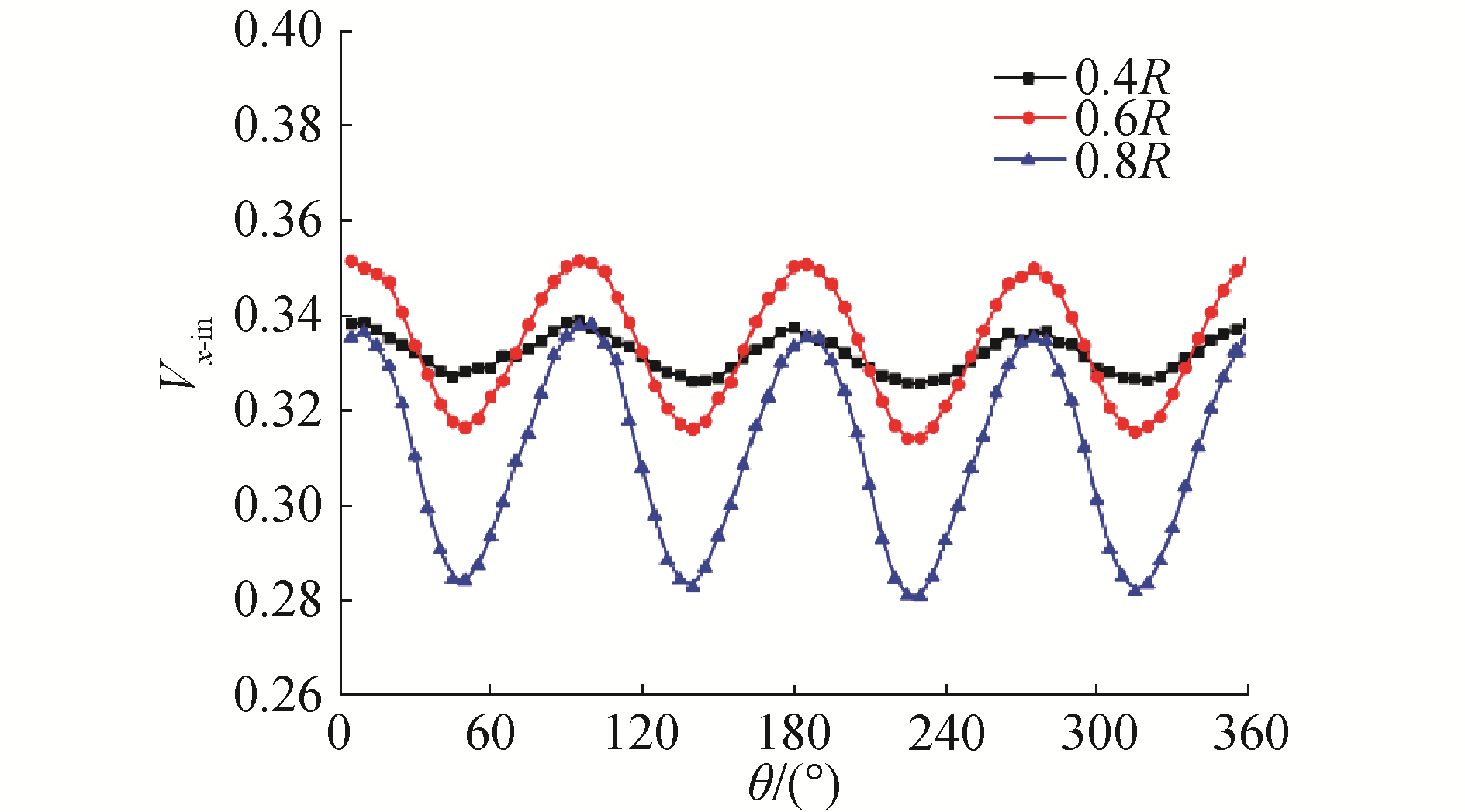Download: 图 3 不同半径处一周内轴向诱导速度分布 Fig. 3 Axial induced velocity profiles at different radii within one circle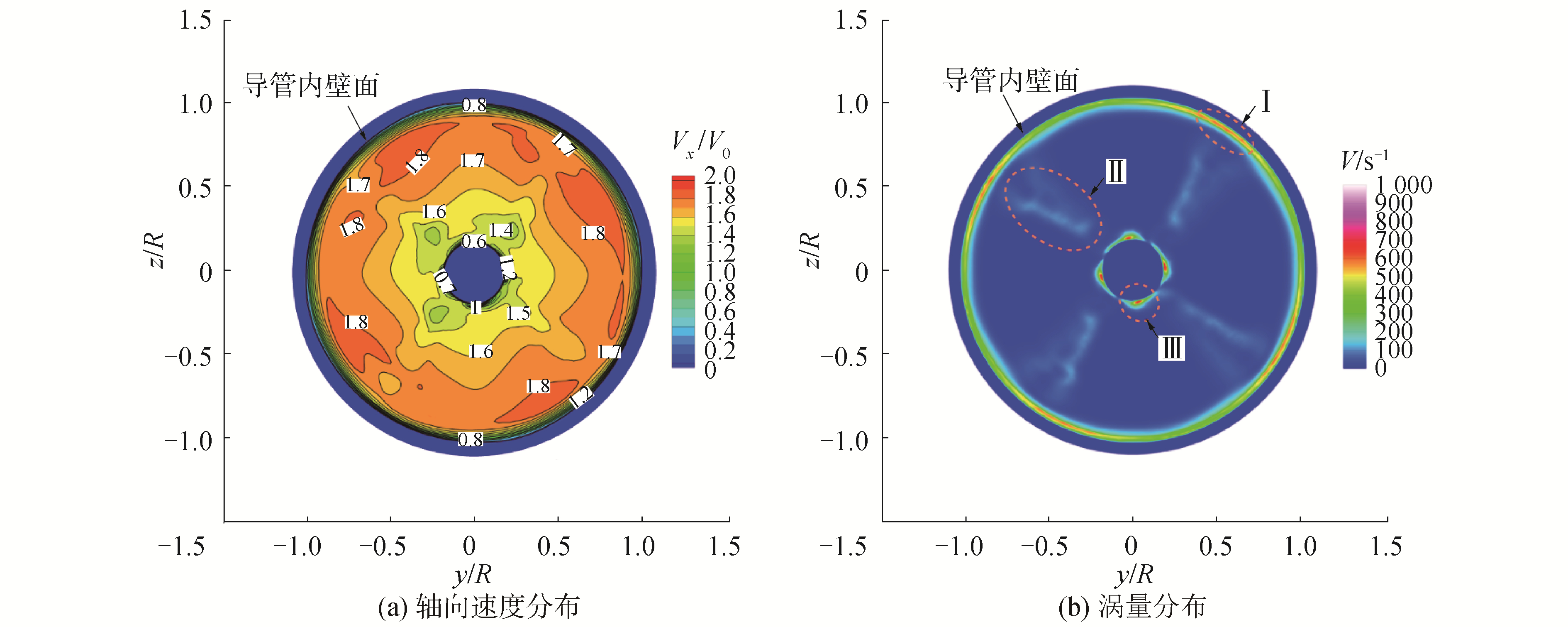Download: 图 4 x=0.3R盘面速度分布 Fig. 4 Velocity of the back disk x=0.3R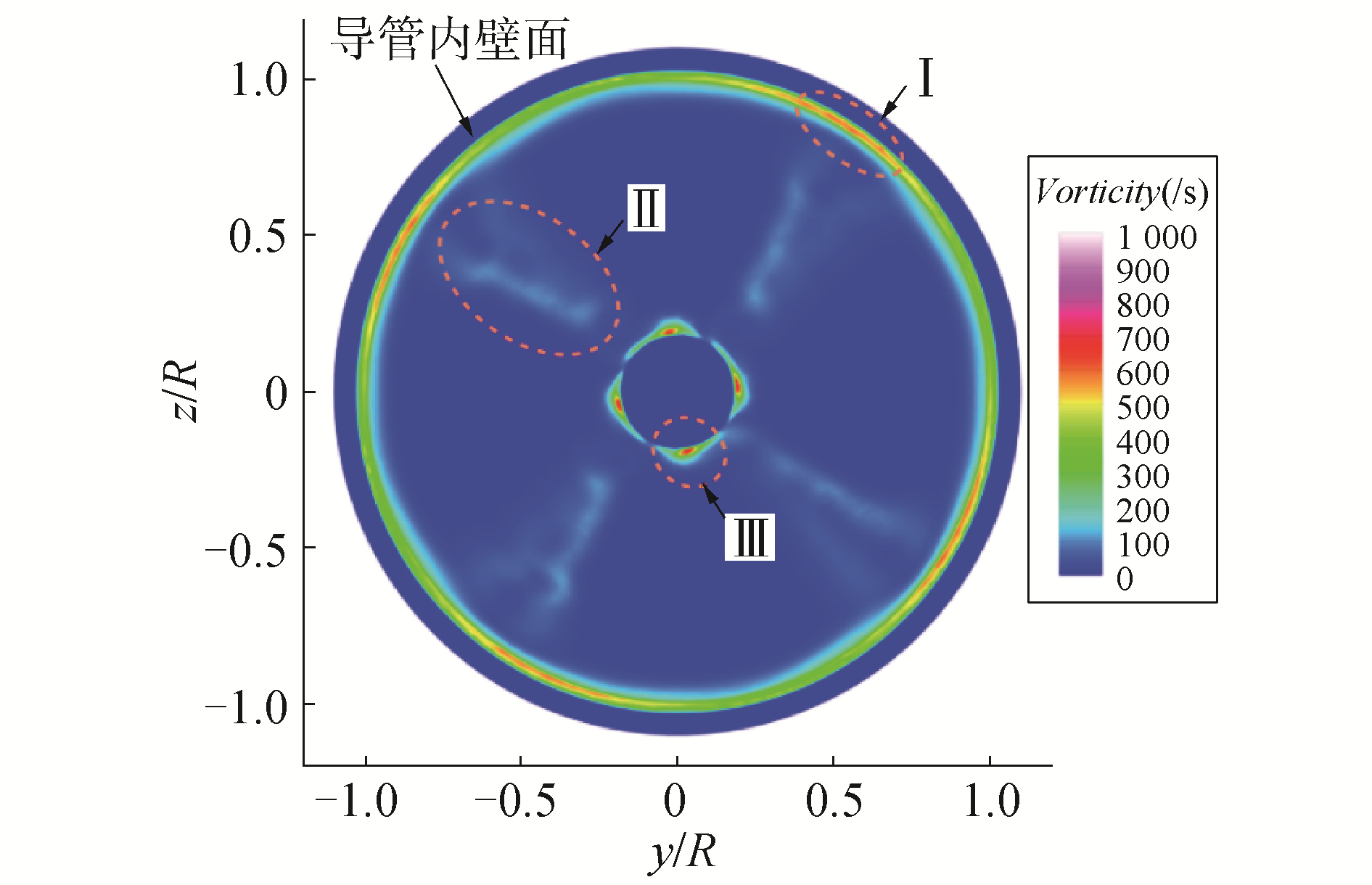Download: 图 5 导管表面瞬态压力分布 Fig. 5 Transient pressure profile of the duct surface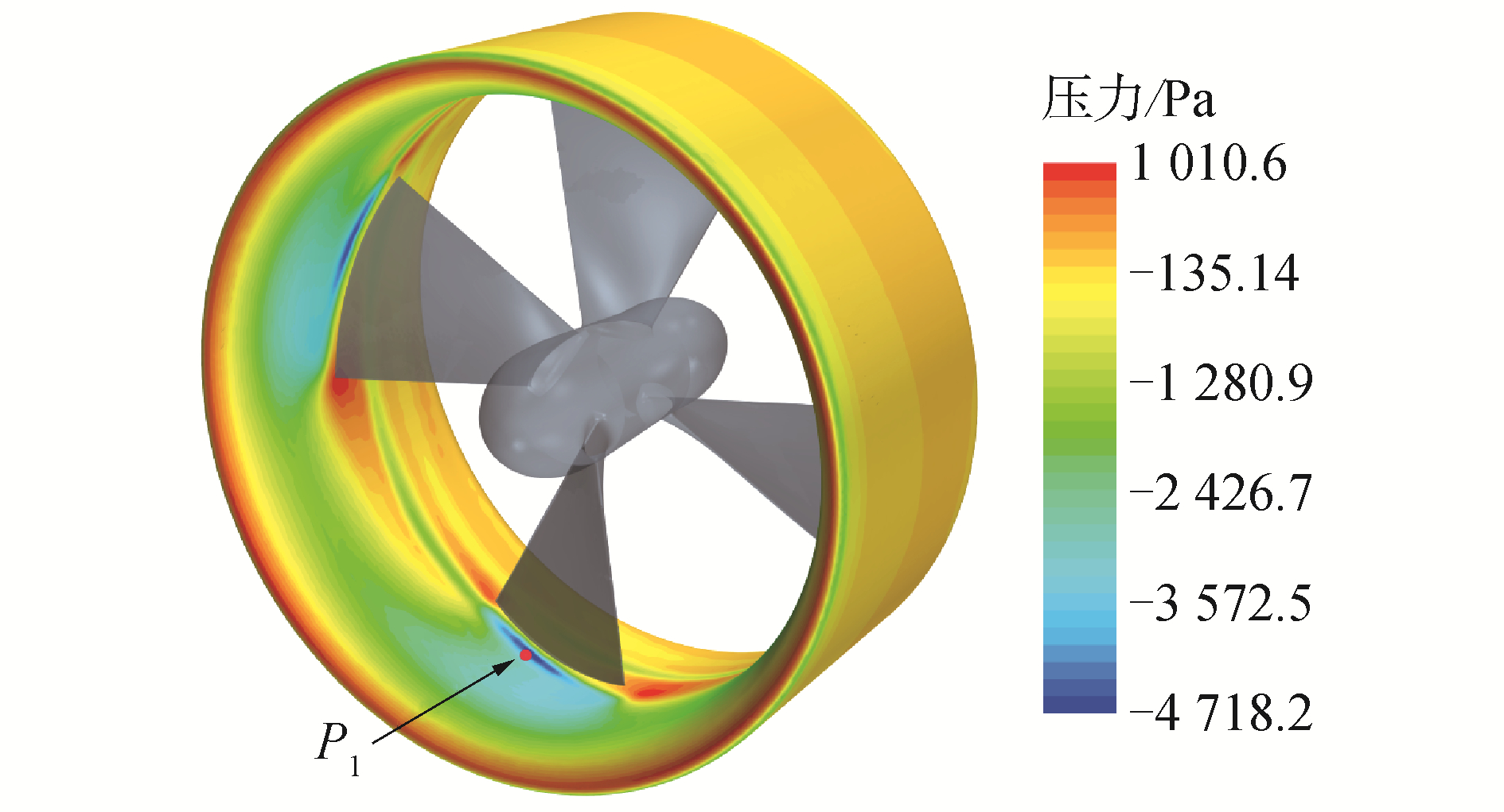Download: 图 6 P1点压力脉动时域图(一个周期内) Fig. 6 Pressure fluctuation in the time domain of probe P1 within one period
3.3 内流场涡特性分析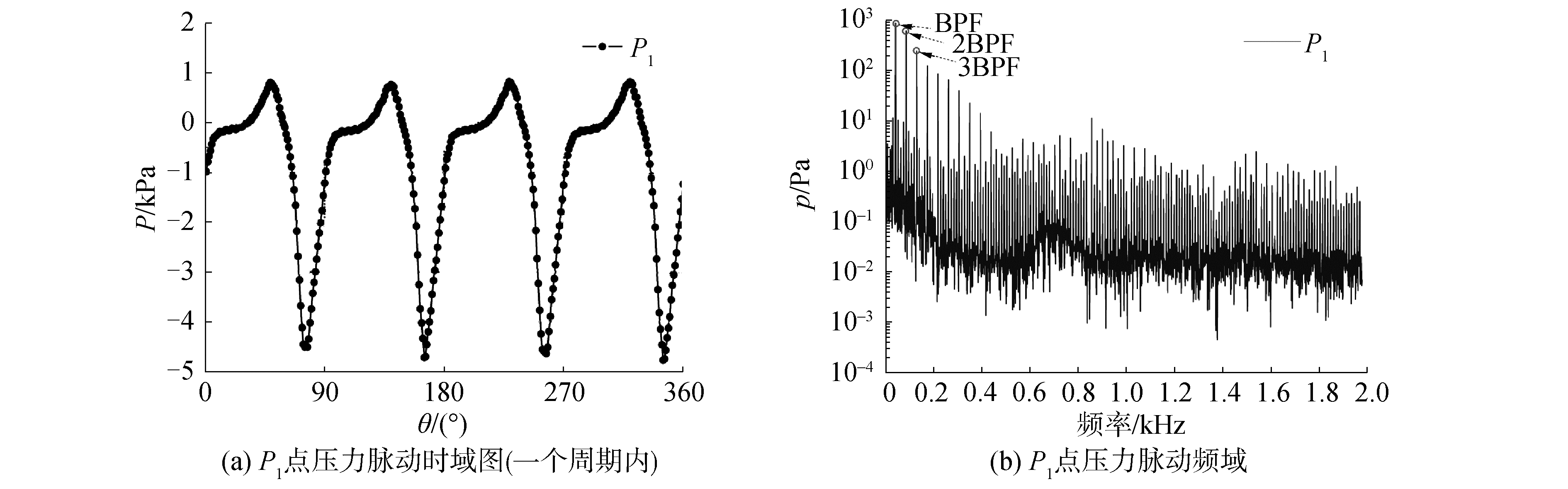Download: 图 7 水平剖面(z=0)内瞬态涡量分布 Fig. 7 Instantaneous vorticity distribution of the horizontal section (z=0)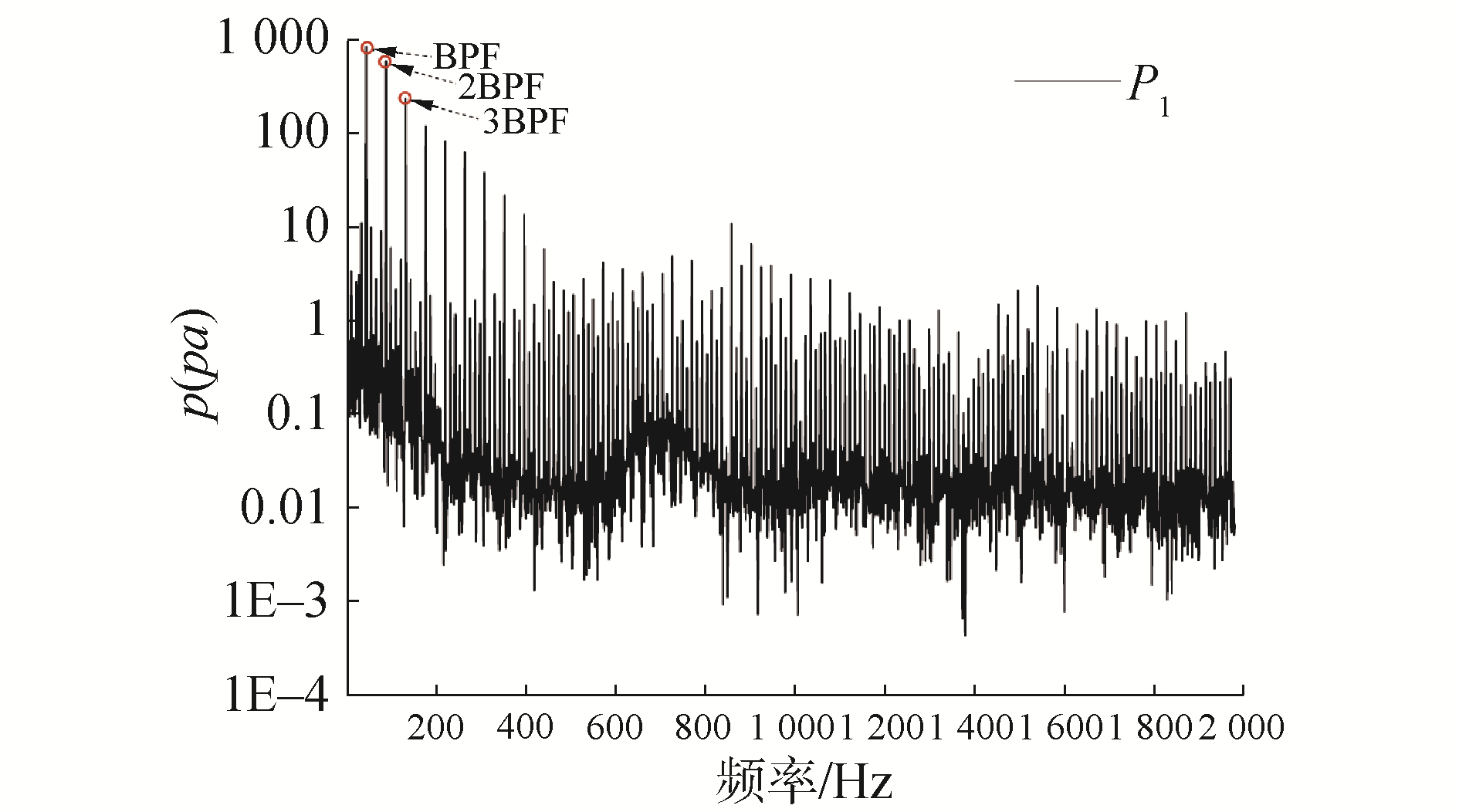Download: 图 8 导管桨内流场三维空间涡量分布结果 Fig. 8 Three-dimensional vorticity distribution in internal flow field of ducted propeller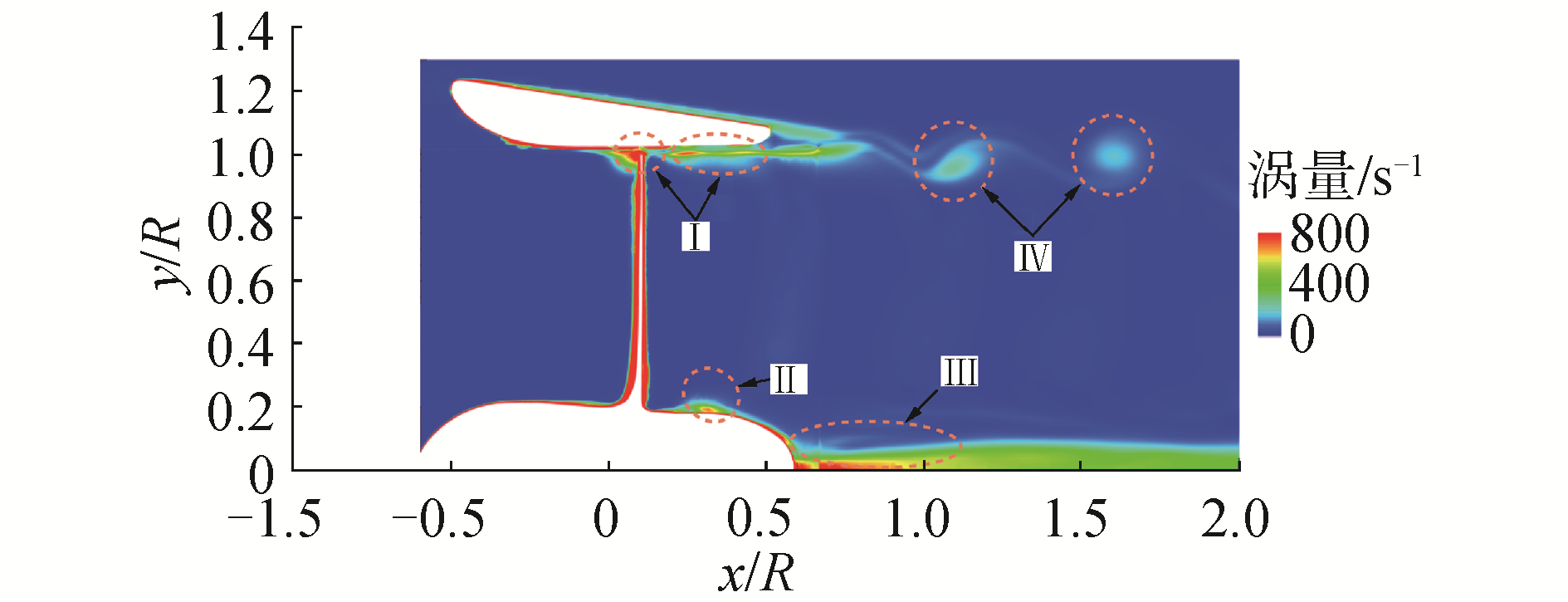Download: 图 9 梢涡结构形态及内部流线结果 Fig. 9 The morphology of the vorticity contour and the internal streamlines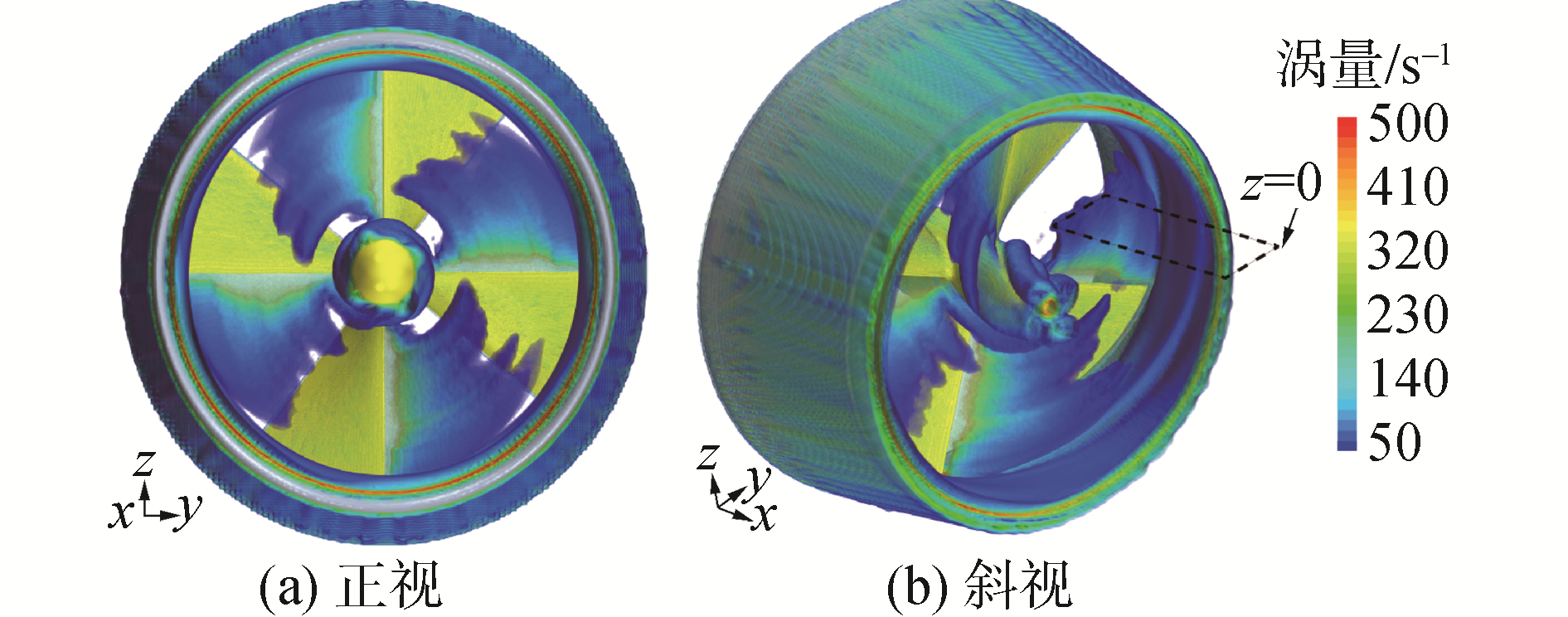Download: 图 10 y/R=1位置处涡量分布 Fig. 10 Vorticity distribution diagram at y/R=1
4 结论

1) 导管内壁区域流动复杂，导管内壁面压力脉动特性与螺旋桨叶频相关；

2) 导管桨尾涡系包含导管剪切层涡、叶梢涡、叶片脱落涡、叶根涡和毂涡，叶片脱落涡向下游传输过程中能量迅速扩散；

3) 导管的存在直接影响螺旋桨梢涡的分布，梢涡形态发生变化，涡量更大面积的分布于导管内壁面，导管有助于螺旋桨尾流的涡能回收从而提高系统推进效率。

  盛振邦, 刘应中. 船舶原理[M]. 上海: 上海交通大学出版社, 2004: 177-188. (0)  王国强, 刘小龙. 用基于速度势的面元法预估导管桨的非定常性能[J]. 船舶力学, 2006, 10(1): 36-42. WANG Guoqiang, LIU Xiaolong. Prediction of unsteady performance of ducted propellers by potential based panel method[J]. Journal of ship mechanics, 2006, 10(1): 36-42. DOI:10.3969/j.issn.1007-7294.2006.01.006 (0)  胡健, 黄胜, 马骋, 等. 影响导管桨内部流场的几个因素[J]. 天津大学学报, 2009, 42(4): 340-344. HU Jian, HUANG Sheng, MA Cheng, et al. Several influence factors for the inner flow field of ducted propeller[J]. Journal of Tianjin University, 2009, 42(4): 340-344. DOI:10.3969/j.issn.0493-2137.2009.04.011 (0)  苏玉民, 刘业宝, 沈海龙, 等. 基于面元法预报导管桨性能的数值计算方法[J]. 华中科技大学学报(自然科学版), 2012, 40(8): 57-61. SU Yumin, LIU Yebao, SHEN Hailong, et al. Surface panel method-based numerical calculation for predicting ducted propeller performances[J]. Journal of Huazhong University of Science and Technology (natural science edition), 2012, 40(8): 57-61. (0)  解学参, 黄胜, 胡健, 等. 导管桨内部流场的数值计算[J]. 哈尔滨工程大学学报, 2009, 30(1): 7-12, 45. XIE Xueshen, HUANG Sheng, HU Jian, et al. Inner flow field calculations for ducted propellers[J]. Journal of Harbin Engineering University, 2009, 30(1): 7-12, 45. DOI:10.3969/j.issn.1006-7043.2009.01.002 (0)  龚杰, 郭春雨, 吴铁成, 等. 基于分离涡模拟方法的导管桨近尾流场及尾涡特性分析[J]. 上海交通大学学报, 2018, 52(6): 674-680. GONG Jie, GUO Chunyu, WU Tiecheng, et al. Detached eddy simulation of near wake field and vortex characteristics for a ducted propeller[J]. Journal of Shanghai Jiao Tong University, 2018, 52(6): 674-680. (0)  刘业宝, 苏玉民, 赵金鑫, 等. 环流理论与泵理论相结合的导管桨设计优化[J]. 哈尔滨工程大学学报, 2014, 35(11): 1307-1313. LIU Yebao, SU Yumin, ZHAO Jinxin, et al. Optimal design of a ducted propeller based on the circulation theory and pump theory[J]. Journal of Harbin Engineering University, 2014, 35(11): 1307-1313. (0)  GONG Jie, GUO Chunyu, ZHAO Dagang, et al. A comparative DES study of wake vortex evolution for ducted and non-ducted propellers[J]. Ocean Engineering, 2018, 160: 78-93. DOI:10.1016/j.oceaneng.2018.04.054 (0)  胡健, 王楠, 胡洋. 加速导管和减速导管的性能比较[J]. 北京航空航天大学学报, 2017, 43(2): 240-252. HU Jian, WANG Nan, HU Yang. Performance comparison of accelerating duct and decelerating duct[J]. Journal of Beijing University of Aeronautics and Astronautics, 2017, 43(2): 240-252. (0)  胡健, 王楠, 胡洋. 导管桨水动力特性的数值研究[J]. 哈尔滨工程大学学报, 2017, 38(6): 815-821. HU Jian, WANG Nan, HU Yang. Numerical study on the hydrodynamic performance of ducted propellers[J]. Journal of Harbin Engineering University, 2017, 38(6): 815-821. (0)  时立攀, 熊鹰, 杨勇, 等. 导管桨周围流场的数值模拟[J]. 哈尔滨工程大学学报, 2016, 37(3): 344-348. SHI Lipan, XIONG Ying, YANG Yong, et al. Numerical simulation of the flow around a ducted propeller using Reynolds-averaged Navier-Stokes equations[J]. Journal of Harbin Engineering University, 2016, 37(3): 344-348. (0)  孙瑜, 苏玉民, 刘伟. 导管结构改进后导管桨的水动力性能研究[J]. 华中科技大学学报(自然科学版), 2016, 44(6): 68-71. SUN Yu, SU Yumin, LIU Wei. Research on hydrodynamic performance of ducted propeller after structure improvement of duct[J]. Journal of Huazhong University of Science and Technology (natural science edition), 2016, 44(6): 68-71. (0)  BHATTACHARYYA A, KRASILNIKOV V, STEEN S. A CFD-based scaling approach for ducted propellers[J]. Ocean engineering, 2016, 123: 116-130. DOI:10.1016/j.oceaneng.2016.06.011 (0)  BHATTACHARYYA A, KRASILNIKOV V, STEEN S. Scale effects on a 4-bladed propeller operating in ducts of different design in open water[C]//Fourth International Symposium on Marine Propulsors smp'15, USA, 2015. (0)  BHATTACHARYYA A, KRASILNIKOV V, STEEN S. Scale effects on open water characteristics of a controllable pitch propeller working within different duct designs[J]. Ocean engineering, 2016, 112: 226-242. DOI:10.1016/j.oceaneng.2015.12.024 (0)  GAGGERO S, TANI G, VIVIANI M, et al. A study on the numerical prediction of propellers cavitating tip vortex[J]. Ocean engineering, 2014, 92: 137-161. DOI:10.1016/j.oceaneng.2014.09.042 (0)  SHUR M L, SPALART P R, STRELETS M K, et al. A hybrid RANS-LES approach with delayed-DES and wall-modelled LES capabilities[J]. International journal of heat and fluid flow, 2008, 29(6): 1638-1649. DOI:10.1016/j.ijheatfluidflow.2008.07.001 (0)  龚杰, 郭春雨, 张海鹏. 喷水推进船模旋转叶轮流场的数值分析[J]. 上海交通大学学报, 2017, 51(3): 326-331. GONG Jie, GUO Chunyu, ZHANG Haipeng. Numerical analysis of impeller flow field of waterjet self-propelled ship model[J]. Journal of Shanghai Jiao Tong University, 2017, 51(3): 326-331. (0)  SPALART P R. Detached-eddy simulation[J]. Annual review of fluid mechanics, 2009, 41: 181-202. DOI:10.1146/annurev.fluid.010908.165130 (0)  DI MASCIO A, MUSCARI R, DUBBIOSO G. On the wake dynamics of a propeller operating in drift[J]. Journal of fluid mechanics, 2014, 754: 263-307. DOI:10.1017/jfm.2014.390 (0)